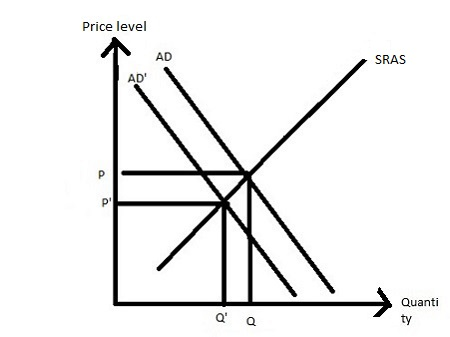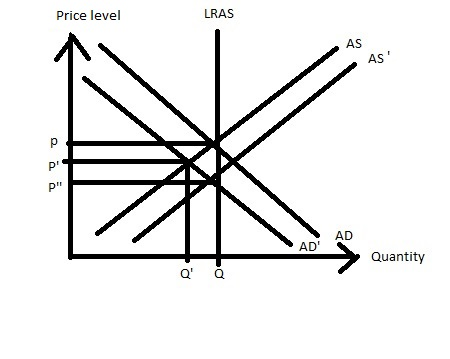# Suppose that the economy is in long-run equilibrium initially, and the Federal Reserve reduces...

## Question:

Suppose that the economy is in long-run equilibrium initially, and the Federal Reserve reduces the money supply. Assume that velocity is constant. Using the quantity theory of money, and the AD-SRAS-LRAS model, explain the following:

A) What happens to aggregate demand?

B) In the short run, how output, prices, and unemployment change?

C) How is the new long-run equilibrium reached? Compare the output level, unemployment, and price level to the short-run equilibrium.

## Aggregate demand and Aggregate supply:

The aggregate demand curve shows the inverse relationship between the price level and total quantity of good demanded such that the aggregate demand curve is downward sloping.

The aggregate supply curve shows the direct relationship between the price level and total quantity of good supplied such that the aggregate supply curve is upward sloping.

## Answer and Explanation:

A) As the federal reserve reduces the money supply then the rate of interest increases such that the investment decreases that causes the aggregate demand curve shifts leftward which decreases both price and output.

B) The federal reserve reduces the money supply in such a way that the aggregate demand curve shifts leftward which reduces both price level and output or unemployment falls.C) As the federal reserve decreases the money supply then the aggregate demand curve shifts leftward and at the same time, the aggregate supply curve shifts rightward such that the price level decreases and quantity remain the same or full employment level of output is achieved.#### Learn more about this topic:Aggregate Supply and Aggregate Demand (AS-AD) Model

from Economics 102: Macroeconomics

Chapter 7 / Lesson 3
58K